# Class 10 RD Sharma Solutions – Chapter 11 Constructions – Exercise 11.2 | Set 2

### Question 11. Construct a triangle similar to a given ΔXYZ with its sides equal to (3/2)th of the corresponding sides of ΔXYZ. Write the steps of construction.

Solution:

Follow these steps for the construction:

Step 1: Construct a triangle XYZ along some feasible data.

Step 2: Construct a ray YL creating an acute angle along XZ and break off 5 equal parts creating

YY1= Y1Y2 = Y2Y3 = Y3Y4.

Step 3: Connect Y4 and Z.

Step 4: From Y3, construct Y3Z’ parallel to Y4Z and Z’X’ parallel to ZX.

Therefore,

We have the required triangle ΔX’YZ’.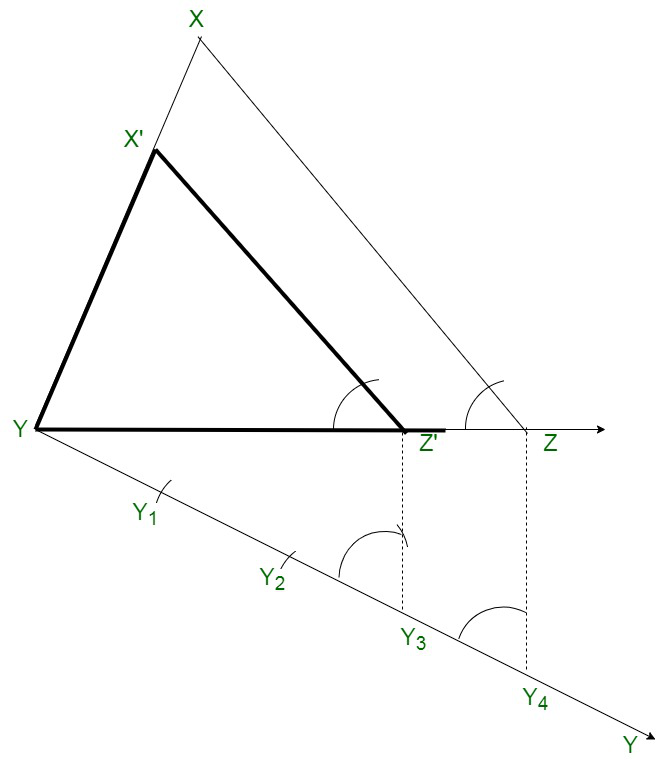### Question 12. Draw a right triangle in which sides (other than the hypotenuse) are of lengths 8 cm and 6 cm. Then construct another triangle whose sides are 3/4 times the corresponding sides of the first triangle.

Solution:

Follow these steps for the construction

Step 1: Construct right ΔABC right angle at B and BC of 8 cm and BA of 6 cm.

Step 2: Construct a line BY creating a cut angle along BC and break off 4 equal parts.

Step 3: Connect 4C and Construct 3C’ || 4C and C’A’ parallel to CA.

Therefore,

We have the required triangle ΔBC’A’ is the required triangle.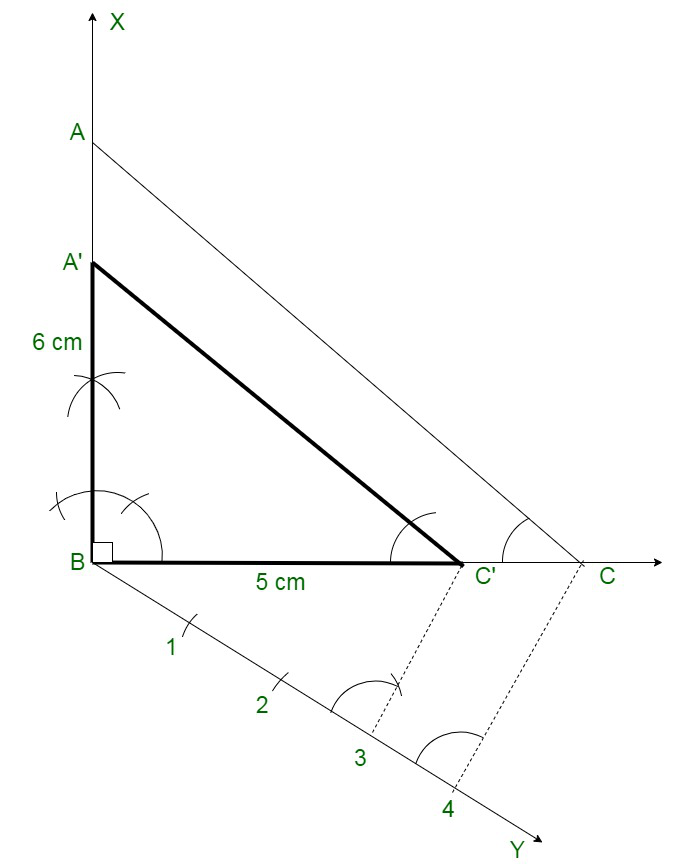### Question 13. Construct a triangle with sides 5 cm, 5.5 cm, and 6.5 cm. Now construct another triangle, whose sides are 3/5 times the corresponding sides of the given triangle.

Solution:

Follow these steps for the construction:

Step 1: Construct a line segment BC of 5.5 cm.

Step 2: Along centre B and radius 5 cm and along centre C and radius 6.5 cm,

Construct arcs that bisect each other at A

Step 3: Connect BA and CA. ΔABC is the given triangle.

Step 4: At B, construct a ray BX creating an acute angle and break off 5 equal parts from BX.

Step 5: Connect C5 and Construct 3D || 5C which connects BC at D.

From D, construct DE || CA which meets AB at E.

Therefore,

We have the required triangle ΔEBD.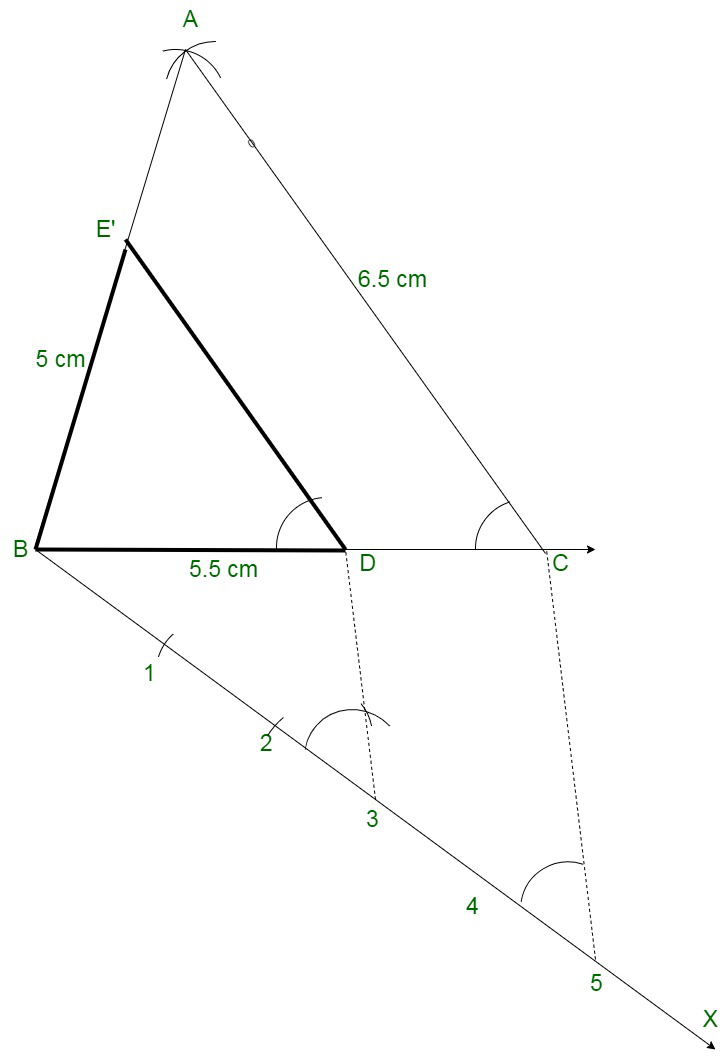### Question 14. Construct a triangle PQR with side QR = 7 cm, PQ = 6 cm and ∠PQR = 60°. Then construct another triangle whose sides are 3/5 of the corresponding sides of ΔPQR.

Solution:

Follow these steps for the construction:

Step 1: Construct a line segment QR = 7 cm.

Step 2: At Q construct a ray QX creating an angle of 60° and cut of PQ = 6 cm.

Connect PR.

Step 3: Construct a ray QY creating an acute angle and break off 5 equal parts.

Step 4: Connect 5, R and through 3, construct 3, S parallel to 5, R which meet QR at S.

Step 5: Through S, construct ST || RP meeting PQ at T.

Therefore,

We have the required ΔQST.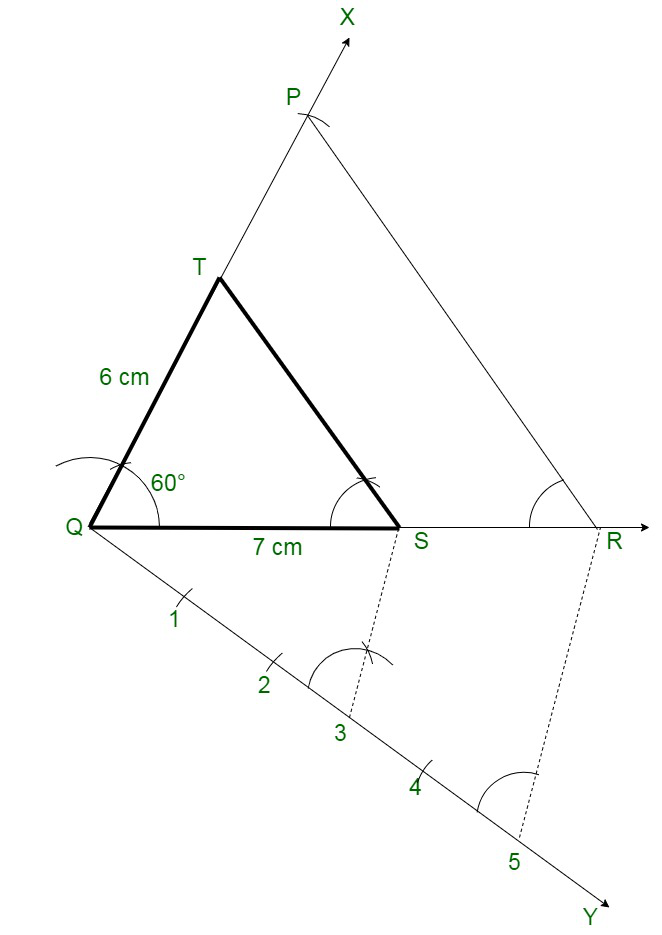### Question 15. Construct a ΔABC in which base BC = 6 cm, AB = 5 cm and ∠ABC = 60°. Then construct another triangle whose sides are 3/4 of the corresponding sides of ΔABC.

Solution:

Follow these steps for the construction:

Step 1: To construct a triangle ABC

Along side of BC of 6 cm,

AB of 5 cm and ∠ABC = 60°.

Step 2: Construct a ray BX, which creates an acute angle ∠CBX below the line BC.

Step 4: Make four points B1, B2, B3 and B4 on BX such that BB1 = B1B2  = B2B3 = B3B4.

Step 5: Connect B4C and construct a line through B3 parallel to B4C bisecting BC to C’.

Step 6: Construct a line through C’ parallel to the line CA to intersect BA at A’.

Therefore,

We have the required triangle ΔABC.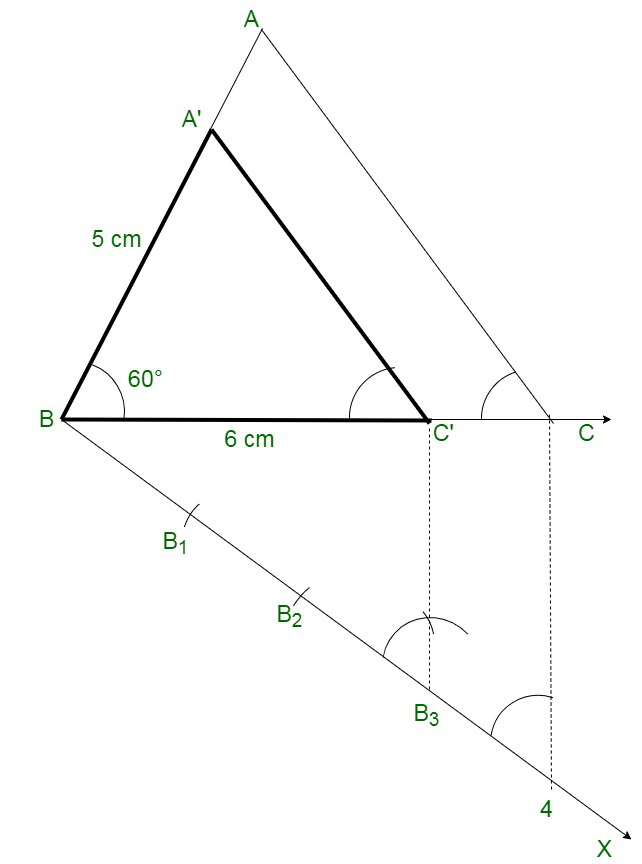### Question 16. Construct a right triangle in which the sides (other than the hypotenuse) arc of lengths 4 cm and 3 cm. Now, construct another triangle whose sides are 5/3 times the corresponding sides of the given triangle.

Solution:

Follow these steps for the construction:

Step 1: Construct a right triangle ABC in which the sides (other than the hypotenuse) are

of lengths 4 cm and 3 cm. ∠B = 90°.

Step 2: Construct a line BX, that creates an acute angle ∠CBX below the line BC.

Step 3: Mark 5 points B1, B22, B3, B4 and B5 on BX such that BB1 = B1B2 = B2B3 = B3B4 = B4B5.

Step 4: Connect B3 to C and Construct a line through B5 parallel to B3C,

bisecting the extended line segment BC at C’.

Step 5: Construct a line through C’ parallel to CA bisecting the extended line segment BA at A’.

Therefore,

We have the required triangle ΔA’BC’.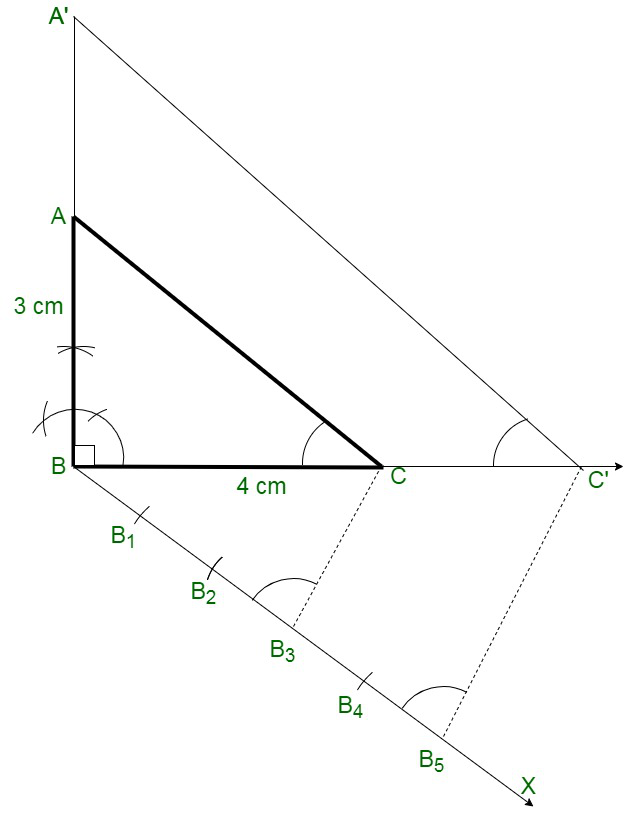### Question 17. Construct a ΔABC in which AB = 5 cm, ∠B = 60°, altitude CD = 3 cm. Construct a ΔAQR similar to ΔABC such that side of ΔAQR is 1.5 times that of the corresponding sides of ΔACB.

Solution:

Follow these steps for the construction:

Step 1: Construct a line segment AB of 5 cm.

Step 2: At A, construct a perpendicular and break off AE = 3 cm.

Step 3: From E, construct EF || AB.

Step 4: From B, construct a ray creating an angle of 60 meeting EF at C.

Step 5: Connect CA. After that ABC is the triangle.

Step 6: From A, construct a ray AX creating an acute angle along AB and break

off 3 equal parts creating AA1 = A1A2 = A2A3.

Step 7: Connect A2 and B.

Step 8: From A , construct A^B’ parallel to A2B and B’C’ parallel to BC.

Therefore,

We have the required triangle ΔC’AB’.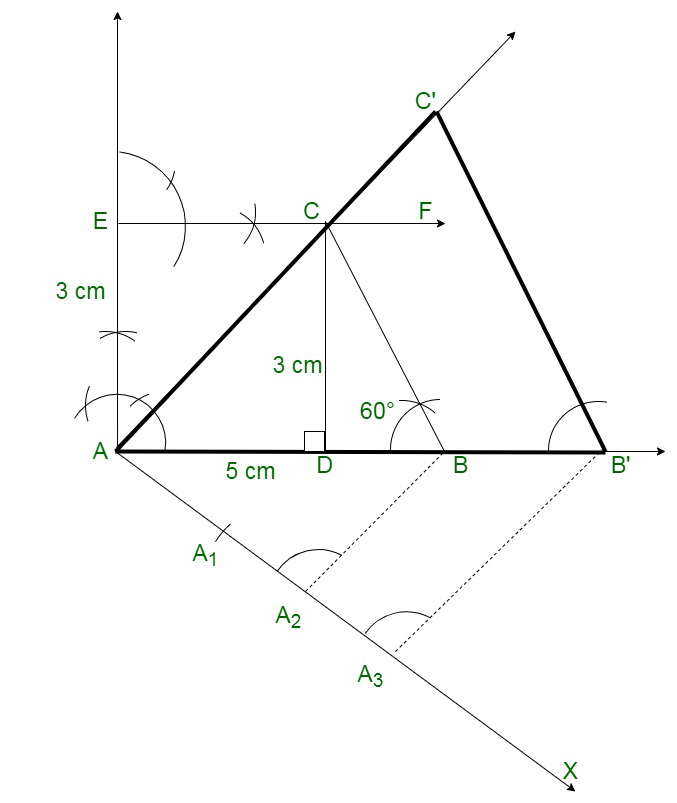Whether you're preparing for your first job interview or aiming to upskill in this ever-evolving tech landscape, GeeksforGeeks Courses are your key to success. We provide top-quality content at affordable prices, all geared towards accelerating your growth in a time-bound manner. Join the millions we've already empowered, and we're here to do the same for you. Don't miss out - check it out now!

Previous
Next